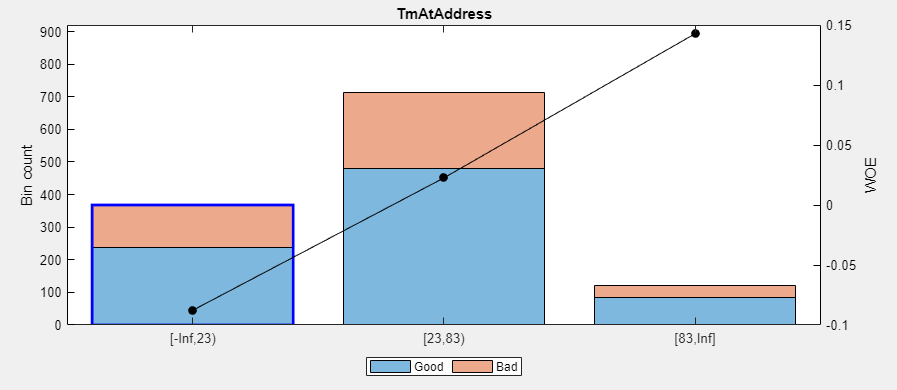Documentation

The Binning Explorer app supports the following tasks:

### Import Data

Binning Explorer enables you to import data by either starting directly from the data stored in a MATLAB® table or by loading an existing `creditscorecard` object.

#### Clean Start from Data

To start directly from data:

1. Place the credit scorecard data in your MATLAB workspace. The data must be in a MATLAB table, where each column of data can be any one of the following data types:

• Numeric

• Logical

• Cell array of character vectors

• Character array

• Categorical

In addition, the table must contain a binary response variable.

2. Open Binning Explorer from the MATLAB toolstrip: On the Apps tab, under Computational Finance, click the app icon.

3. Select the data from the Step 1 pane of the Import Data window.

4. From the Step 2 pane, set the Variable Type for each of the predictors, as needed. If the input MATLAB table contains a column for `weights`, from the Step 2 pane, using the Variable Type column, click the drop-down to select Weights. If the data contains missing values, from the Step 2 pane, set Bin missing data: to Yes. For more information on working with missing data, see Credit Scorecard Modeling with Missing Values (Financial Toolbox).

5. From the Step 3 pane, select an initial binning algorithm and click . The bins are plotted and displayed for each predictor. By clicking an individual predictor plot, the details for that predictor plot display in the Bin Information and Predictor Information panes.

#### Start from an Existing `creditscorecard` Object

To start using an existing `creditscorecard` object:

1. Place the `creditscorecard` object in your MATLAB workspace. Create the `creditscorecard` object either by using `creditscorecard` or by clicking in the Binning Explorer to export and save a `creditscorecard` object to the MATLAB workspace.

2. Open Binning Explorer from the MATLAB toolstrip: On the Apps tab, under Computational Finance, click the app icon.

3. From Step 1 pane of the Import Data window, select the `creditscorecard` object.

4. From the Step 3 pane, select a binning algorithm. When using an existing `creditscorecard` object, it is recommended to select the option. To display the predictor plots, click .

The bins are plotted and displayed for each predictor. By clicking an individual predictor plot, the details for that predictor plot display in the Bin Information and Predictor Information panes.

#### Start from MATLAB Command Line Using Data or an Existing `creditscorecard` Object

To start Binning Explorer from the MATLAB command line:

1. Place the credit scorecard data or existing `creditscorecard` object in your MATLAB workspace.

2. At the MATLAB command prompt:

• Enter `binningExplorer(data)` or `binningExplorer(data,Name,Value)` to open a table in the Binning Explorer app by specifying a table (`data`) as input.

• Enter `binningExplorer(sc)` to open an existing `creditscorecard` object in the Binning Explorer app by specifying a `creditscorecard` object (`sc`) as input.

The bins are plotted and displayed for each predictor. By clicking an individual predictor plot, the details for that predictor plot display in the Bin Information and Predictor Information panes.

### Change Predictor Type

After you import data or a `creditscorecard` object into Binning Explorer, you can change the predictor type.

1. Click any predictor plot. The name of the selected predictor displays on the Binning Explorer toolstrip under Selected Predictor.

On the Binning Explorer toolstrip, the predictor type for the selected predictor displays under Predictor Type.

2. To change the predictor type, under Predictor Type, select: `Numeric`, `Categorical`, or `Ordinal`. The predictor plot is updated and the details in the Bin Information and Predictor Information panes are also updated.

### Change Binning Algorithm for One or More Predictors

After you import data or a `creditscorecard` object into Binning Explorer, you can change the binning algorithm for an individual predictor or for multiple predictors.

1. Click any predictor plot. The selected predictor plot displays with a blue outline.### Tip

When you select a predictor plot with the blue outline, a status message appears at the bottom of the Binning Explorer that displays the last binning information for that predictor. Use this information to determine which binning algorithm is most recently applied to an individual predictor plot.

2. On the Binning Explorer toolstrip, click Apply Monotone and select Monotone, Split, Merge, Equal Frequency, or Equal Width. The predictor plot is updated with a change of algorithm. The details in the Bin Information and Predictor Information panes are also updated.

3. To change the binning algorithm for multiple predictors, multiselect more than one predictor plot by using Ctrl + click to highlight each predictor plot with a blue outline.4. Click Apply Monotone and select Monotone, Split, Merge, Equal Frequency, or Equal Width. All the selected predictor plots are updated for a change of algorithm.

### Change Algorithm Options for Binning Algorithms

After you import data or a `creditscorecard` object into Binning Explorer, you can change the binning algorithm options for an individual predictor or for multiple predictors.

1. Click any predictor plot. The predictor plot displays with a blue outline.### Tip

When you select a predictor plot with the blue outline, a status message appears at the bottom of the Binning Explorer that displays the last binning information for that predictor. Use this information to determine which binning algorithm is most recently applied to an individual predictor plot.

2. On the Binning Explorer toolstrip, click to open the Algorithm Options dialog box.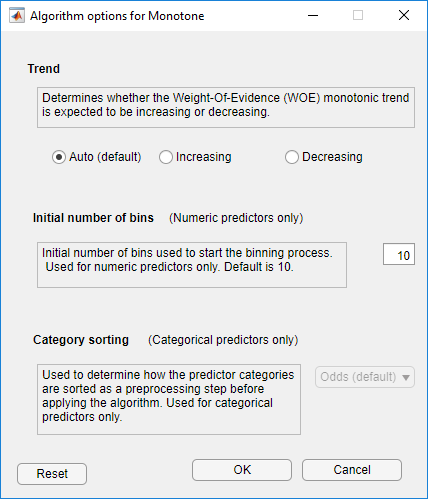3. From the associated Algorithm options dialog box:

• Monotone

• For Trend, select one of the following:

• Auto (default) — Automatically determines if the WOE trend is increasing or decreasing.

• Increasing — Looks for an increasing WOE trend.

• Decreasing — Looks for a decreasing WOE trend.

The value of Trend does not necessarily reflect that of the resulting WOE curve. The Trend option tells the algorithm to look for an increasing or decreasing trend, but the outcome might not show the desired trend. For example, the algorithm cannot find a decreasing trend when the data actually has an increasing WOE trend. For more information on the Trend option, see Monotone (Financial Toolbox).

• For Initial number of bins, enter an initial number of bins (default is `10`). The initial number of bins must be an integer > `2`. Used for numeric predictors only.

• For Category Sorting, used for categorical predictors only, select one of the following:

• Odds (default) — The categories are sorted by order of increasing values of odds, defined as the ratio of “Good” to “Bad” observations, for the given category.

• Goods — The categories are sorted by order of increasing values of “Good.”

• Bads — The categories are sorted by order of increasing values of “Bad.”

• Totals — The categories are sorted by order of increasing values of the total number of observations (“Good” plus “Bad”).

• None — No sorting is applied. The existing order of the categories is unchanged before applying the algorithm.

• Split

• For Measure, select one of the following: Gini (default), Chi2, InfoValue, or Entropy.

• For Tolerance, specify a tolerance value above which the gain in the information value has to be for the split to be accepted. The default is `1e-4`.

• For Significance, only for the Chi2 measure, specify a significance level threshold for the chi-square statistic, above which splitting happens. Values are in the interval `[0,1]`. Default is `0.9` (90% significance level).

• For Bin distribution, specify values for

• MinBad — Specifies the minimum number n (n>=`0`) of Bads per bin. The default value is `1`, to avoid pure bins.

• MaxBad — Specifies the maximum number n (n>=`0`) of Bads per bin. The default value is `Inf`.

• MinGood — Specifies the minimum number n (n>=`0`) of Goods per bin. The default value is `1`, to avoid pure bins.

• MaxGood — Specifies the maximum number n (n>=`0`) of Goods per bin. The default value is `Inf`.

• MinCount — Specifies the minimum number n (n>=`0`) of observations per bin. The default value is `1`, to avoid empty bins.

• MaxCount — Specifies the maximum number n (n>=`0`) of observations per bin. The default value is `Inf`.

• MaxNumBins — Specifies the maximum number n (n>=`2`) of bins resulting from the splitting. The default value is `5`.

• For Initial number bins, specify an integer that determines the number (n >0) of bins that the predictor is initially binned into before splitting. Valid for numeric predictors only. Default is `50`.

• For Category sorting, used for categorical predictors only, select a value:

• Goods — The categories are sorted by order of increasing values of “Good.”

• Bads — The categories are sorted by order of increasing values of “Bad.”

• Odds — (default) The categories are sorted by order of increasing values of odds, defined as the ratio of “Good” to “Bad” observations, for the given category.

• Totals — The categories are sorted by order of increasing values of total number of observations (“Good” plus “Bad”).

• None — No sorting is applied. The existing order of the categories is unchanged before applying the algorithm. (The existing order of the categories can be seen in the category grouping optional output from `bininfo`.)

• Merge

• For Measure, select one of the following: Chi2 (default), Gini, InfoValue, or Entropy.

• For Tolerance, specify the minimum threshold below which merging happens for the information value and entropy statistics. Valid values are in the interval `(0.1)`. Default is `1e-3`.

• For Significance, specify the significance level threshold for the chi-square statistic, below which merging happens. Values are in the interval `[0,1]`. Default is `0.9` (90% significance level).

• For Bin distribution, specify the following:

• MinNumBins — Specifies the minimum number n (n>=`2`) of bins that result from merging. The default value is `2`.

• MaxNumBins — Specifies the maximum number n (n>=`2`) of bins that result from merging. The default value is `5`.

• For Initial number of bins, specify an integer that determines the number (n >0) of bins that the predictor is initially binned into before merging. Valid for numeric predictors only. Default is `50`.

• For Category sorting, used for categorical predictors only. Select a value:

• Goods — The categories are sorted by order of increasing values of “Good.”

• Bads — The categories are sorted by order of increasing values of “Bad.”

• Odds — (default) The categories are sorted by order of increasing values of odds, defined as the ratio of “Good” to “Bad” observations, for the given category.

• Totals — The categories are sorted by order of increasing values of total number of observations (“Good” plus “Bad”).

• None — No sorting is applied. The existing order of the categories is unchanged before applying the algorithm. (The existing order of the categories can be seen in the category grouping optional output from `bininfo`.)

• Equal Frequency

• For Number of bins, enter the number of bins. The default is `5`, and the number of bins must be a positive number.

• For Category Sorting, select one of the following:

• Odds (default) — The categories are sorted by order of increasing values of odds, defined as the ratio of “Good” to “Bad” observations, for the given category.

• Goods — The categories are sorted by order of increasing values of “Good.”

• Bads — The categories are sorted by order of increasing values of “Bad.”

• Totals — The categories are sorted by order of increasing values of the total number of observations (“Good” plus “Bad”).

• None — No sorting is applied. The existing order of the categories is unchanged before applying the algorithm.

### Note

You can use Category Sorting with categorical predictors only.

• Equal Width

• For Number of bins, enter the number of bins. The default is `5` and the number of bins must be a positive number.

• For Category Sorting, select one of the following:

• Odds (default) — The categories are sorted by order of increasing values of odds, defined as the ratio of “Good” to “Bad” observations, for the given category.

• Goods — The categories are sorted by order of increasing values of “Good.”

• Bads — The categories are sorted by order of increasing values of “Bad.”

• Totals — The categories are sorted by order of increasing values of the total number of observations (“Good” plus “Bad”).

• None — No sorting is applied. The existing order of the categories is unchanged before applying the algorithm.

### Note

You can use Category Sorting with categorical predictors only.

Click . The predictor plot is updated with the change of algorithm options. The details in the Bin Information and Predictor Information panes are also updated.

4. To change the binning algorithm option for multiple predictors, multiselect more than one predictor plot by using Ctrl+ click to highlight each predictor plot with a blue outline.5. On the Binning Explorer toolstrip, click to open the Algorithm Options dialog box. Make your selection from the Algorithm Options dialog box and click . The selected predictor plots are updated for the change of algorithm.

### Split Bins for a Numeric Predictor

After you import data or a `creditscorecard` object into Binning Explorer, you can split bins for a numeric predictor.

1. Click any numeric predictor plot. The predictor plot displays with a blue outline.2. On the Binning Explorer toolstrip, click to open the selected numeric predictor in a new tabbed window.

3. Click a bin to enable the button for that bin.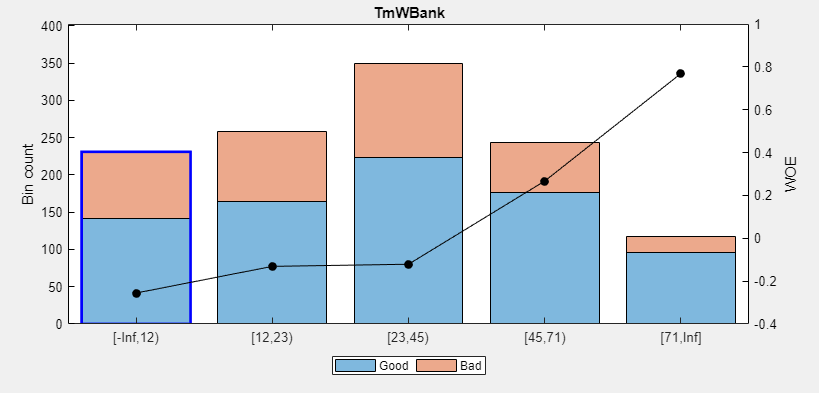### Note

The button is enabled when the data range of the selected bin has more than one value.

4. On the Binning Explorer toolstrip, the Edges text boxes display values for the edges of the selected bin. Click to open the Split dialog box.5. Use the Number of bins control to split the selected bin into multiple bins. Click to complete the split operation.

The plot for the selected numeric predictor is updated with the new bin information. The details in the Bin Information and Predictor Information panes are also updated.

### Split Bins for a Categorical Predictor

After you import data or a `creditscorecard` object into Binning Explorer, you can split bins for a categorical predictor.

1. Click any categorical predictor plot. The predictor plot displays with a blue outline.2. On the Binning Explorer toolstrip, click to open the selected categorical predictor in a new tabbed window.

3. Click a bin to enable the button for that bin.### Note

The button is enabled when the selected bin has more than one category in it.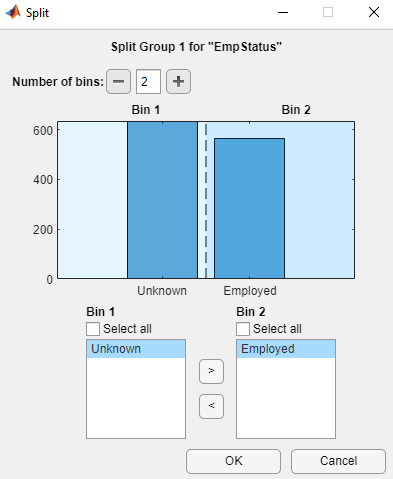Use the Number of bins control to split the selected bin into multiple bins.

Use the arrow controls on the Split dialog box to control the contents for each of the bins that you are splitting the selected bin into.

4. Click to complete the split operation.

The plot for the selected categorical predictor is updated with the new bin information. The details in the Bin Information and Predictor Information panes are also updated.

### Manual Binning to Merge Bins for a Numeric or Categorical Predictor

After you import data or a `creditscorecard` object into Binning Explorer, you can split or merge bins for a predictor.

1. Click any predictor plot. The predictor plot displays with a blue outline.2. On the Binning Explorer toolstrip, click to open the selected predictor in a new tabbed window.

### Note

The button is active only when more than one bin is selected. Only adjacent bins can be merged for numeric or ordinal predictors. Nonadjacent bins can be merged for categorical predictors.

3. To merge bins, select two or more bins for merging by using Ctrl + click to multiselect bins to display with blue outlines.When performing a merge with a numeric predictor, the Edges text boxes on the Binning Explorer toolstrip display the values for the edges of the selected bins to merge.

4. Click to complete the merge operation. The plot for the selected predictor is updated with the new bin information. The details in the Bin Information and Predictor Information panes are also updated.

### Change Bin Boundaries for a Single Predictor

After you import data or a `creditscorecard` object into Binning Explorer, you can change the bin boundaries for a single predictor.

1. Click any predictor plot. The predictor plot displays with a blue outline.2. On the Binning Explorer toolstrip, click . Click to select a specific bin where you want to change the bin dimensions. The selected bin displays with a blue outline.3. On the Binning Explorer toolstrip, the Edges text boxes display values for the edges of the selected bin.Edit the values in the Edges text boxes to change the selected bin’s dimensions.

4. Press Enter to complete the operation. The plot for the selected predictor is updated with the updated bin’s dimension information. The details in the Bin Information and Predictor Information panes are also updated.

### Change Bin Boundaries for Multiple Predictors

After you import data or a `creditscorecard` object into Binning Explorer, you can change the algorithm applied to one or more predictors and you can also redefine the number of bins.

1. Click any predictor plot. The predictor plot displays with a blue outline.Alternatively, select two or more predictors by using Ctrl + click to multiselect predictors to display with blue outlines.2. On the Binning Explorer toolstrip, click to open the Algorithm Options dialog box.

3. The Algorithm Options dialog box displays the options for the current binning algorithm. Depending on which is the current algorithm, you can change bin boundaries:

• If your current algorithm for the selected bins is `EqualWidth` or `EqualFrequency`, enter a number in the Number of bins box. Optionally, for `EqualWidth` and `EqualFrequency` options, under Category Sorting, specify the type of sorting.

• If your current algorithm for the selected bins is `Monotone`, `Split`, or `Merge` the default of `10` for `Monotone` or `50` for `Split` and `Merge` is used for the Initial number of bins. Optionally, for `Monotone`, you can set values for Trend and Category Sorting.

4. Click to complete the operation. The plots for the selected predictors are updated with the new bin information. The details in the Bin Information and Predictor Information panes are also updated.

### Set Options for Display

Binning Explorer has options for displaying predictor plots and plot options and the associated tables displayed in Bin Information.

#### Plot Options

1. From the Binning Explorer toolstrip item for , select any of the following predictor plot options:

• No labels (default)

• Bin count

• % Bin level

• % Data level

• % Total count

• WOE curve

2. The selected label is applied to all predictor plots.

#### Table Options

You can set the table display options for predictor information displayed in Bin Information.

1. From the Binning Explorer toolstrip item for , select any of the following options:

• Odds

• WOE

• InfoValue

• Entropy

• Chi2

• Gini

• Members (option is enabled for categorical predictors)

2. When selected, these options are applied to all predictors for the information displayed in Bin Information.

### Export and Save the Binning

Binning Explorer enables you to export and save your credit scorecard binning definitions to a `creditscorecard` object.

1. Click and provide a `creditscorecard` object name. The `creditscorecard` object is saved to the MATLAB workspace.

### Note

If you export a previously existing `creditscorecard` object that was fit (using `fitmodel`), all fitting settings in the `creditscorecard` object are lost. You must rerun `fitmodel` on the updated `creditscorecard` object.

2. To reopen a previously saved `creditscorecard` object, click and select the `creditscorecard` object from the Step 1 pane of the Import Data window.

### Troubleshoot the Binning

This topic shows some of the results when using Binning Explorer with credit scorecards that need troubleshooting. For details on the overall process of creating and developing credit scorecards, see Overview of Binning Explorer and Binning Explorer Case Study Example.

#### Numeric Predictor Converted to Categorical Predictor Does Not Display Split Data Properly

When you convert a numeric predictor with hundreds of values (for example, continuous data) to categorical data, the resulting data has hundreds of categories. The following example illustrates this scenario.

```load CreditCardData ```

Open the Binning Explorer and select the numeric predictor AMBalance. From the Binning Explorer toolstrip, change the predictor type to Categorical.

Select Manual Binning on the Binning Explorer toolstrip and click . The Split dialog box displays as follows:The predictor has too many categories to display properly.

Solution: If you have a categorical predictor with a large number of categories, use the Algorithm Options to change the binning algorithm for that predictor to Equal Frequency, with the Number of bins set to `100` (or another smaller value). The Split dialog box then displays properly.#### Predictor Plot Appears Distorted

When using the Binning Explorer, if you import data that has not been previously binned and you select from the Import Data window, the resulting plots might be distorted. For example, if you load the following data set into the MATLAB workspace and use Binning Explorer to import the data using , the following plot displays for the TmAtAddress predictor.

```load CreditCardData ```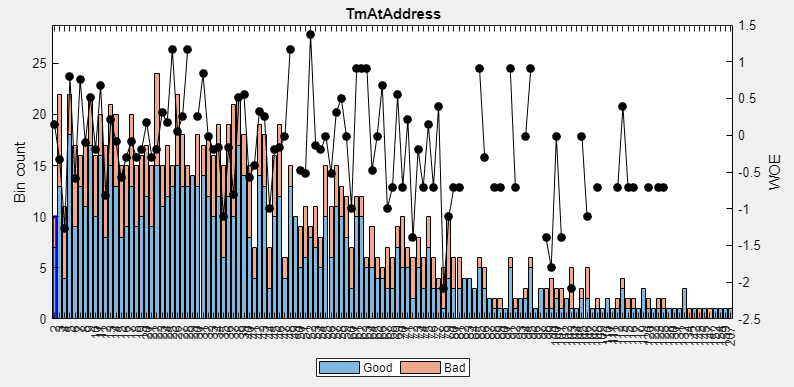Solution: When you import data that has not been previously binned, select from the Import Data window instead. The following plot displays for the TmAtAddress predictor.